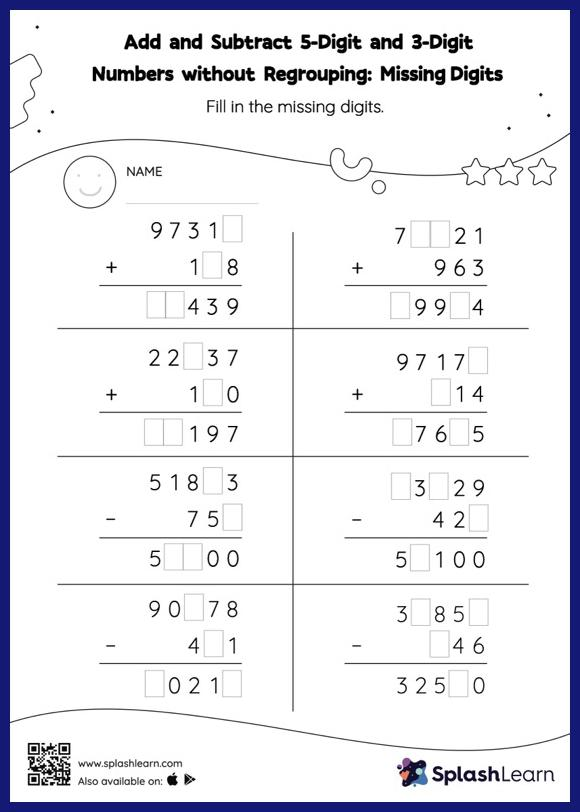# Add and Subtract 5-Digit and 3-Digit Numbers without Regrouping: Missing Digits Worksheet

Home > Add and Subtract 5-Digit and 3-Digit Numbers without Regrouping: Missing DigitsTo find the missing number on this worksheet, students can utilize the connection between addition and subtraction. In add and subtract 5-digit and 3-digit numbers without regrouping worksheet, they do not need to regroup numbers to reach the solution. As the worksheet uses the column method, it is helpful in getting students toward higher accuracy, especially with bigger numbers and in scenarios where regrouping is required.